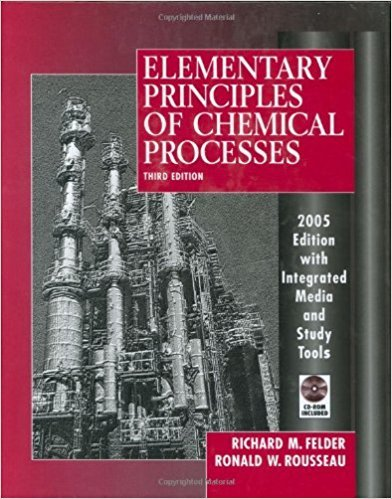×
Get Full Access to Elementary Principles Of Chemical Processes - 3 Edition - Chapter 9 - Problem 9.1
Get Full Access to Elementary Principles Of Chemical Processes - 3 Edition - Chapter 9 - Problem 9.1

×

# The standard heat of the reaction IS t::.li~ = -904.7ISBN: 9780471687573 143

## Solution for problem 9.1 Chapter 9

Elementary Principles of Chemical Processes | 3rd Edition

• Textbook Solutions
• 2901 Step-by-step solutions solved by professors and subject experts
• Get 24/7 help from StudySoup virtual teaching assistantsElementary Principles of Chemical Processes | 3rd Edition

4 5 1 365 Reviews
11
0
Problem 9.1

The standard heat of the reaction IS t::.li~ = -904.7 kllmol (a) Briefly explain what that means. Your explanation may take the form "When (specify quantities of reactant species and their physical states) react to form (quantities of product species and their physical state), the change in enthalpy is ." (b) Is the reaction exothermic or endothermic at 2S C C? Would you have to heat or cool the reactor to keep the temperature constant? What would the temperature do if the reactor ran adiabatically? What can you infer about the energy required to break the molecular bonds of the reactants and that released when the product bonds form? 476 Chapter 9 Balances on Reactive Processes (c) What is D.ii~ for 2 NH3(g) + Oz -- 2 NO(g) + 3 HzO(g) (d) What is D.ii~ for NO(g) + HzO(g) -----+ NH3 (g) + Oz (e) Estimate the enthalpy change associated with the consumption of 340 g NH3/s if the reactants and products are all at 25C. (See Example 9.1-1.) What have you assumed about the reactor pressure? (You don't have to assume that it equals 1 atm.) (0 The values of Mf~ given in this problem apply to water vapor at 25C and 1 atm, and yet the normal boiling point of water is 100C. Can water exist as a vapor at 25C and a total pressure of 1 atm? Explain your answer

Step-by-Step Solution:
Step 1 of 3

14.4 THE CHANGE OF CONCENTRATION WITH TIME First-Order Reactions - first-order reaction – one whose rate depends on the concentration of a single reactant raised to the first power - if the rxn of the type A products is first order, the rate law is Rate = - ∆ A = k [A] ∆t - this form, which expresses how rate depends on concentration, is called differential rate law - this relationship can be transformed into an eqn known as integrated rate law for a first-order rxn that relates the initial concentration of A, [A] 0 to its concentration at any other time t, [A] t ln[A] t ln[A] =0-k t or

Step 2 of 3

Step 3 of 3

##### ISBN: 9780471687573

Unlock Textbook Solution